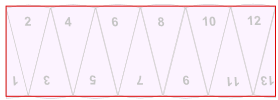# Area of a Circle by Cutting into Sectors

Here is a way to find the formula for the area of a circle:Cut a circle into equal sectors (12 in this example)

Divide just one of the sectors into two equal parts. We now have thirteen sectors – number them 1 to 13:Rearrange the 13 sectors like this:Which resembles a rectangle:What are the (approximate) height and width of the rectangle?

The height is the circle's radius: just look at sectors 1 and 13 above. When they were in the circle they were "radius" high.

The width (actually one "bumpy" edge) is half of the curved parts around the circle ... in other words it is about half the circumference of the circle.

We know that:

Circumference = 2 × π × radius

And so the width is about:

Half the Circumference = π × radius

And so we have (approximately):radius π × radius

Now we just multply the width by the height to find the area of the rectangle: